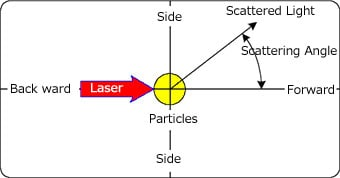# Fraunhofer Diffraction Theory and Mie Scattering Theory

With the laser diffraction/scattering method, particle size is specified from the light intensity distribution pattern. For this, the correspondence relationship between particle size and light intensity distribution pattern must be known in advance. The Fraunhofer diffraction theory and Mie scattering theory are used to obtain this relationship. That is, these theories are used to calculate what kind of light intensity distribution patterns are produced by particles of various sizes, and this data is stored beforehand on a computer as a parameter table (numerical table) containing a vast amount of information.

A considerable amount of time is required for calculating this parameter table. However, in actual measurement of particle size distribution, the measurement time is not affected at all since there are parameter tables already calculated and stored in computer memory.

Ok, then. Let's take a look at the relationship between the Fraunhofer diffraction theory and Mie scattering theory.

In a word, the Fraunhofer diffraction theory is one of the approximate expressions of the Mie scattering theory. This approximate expression can be used only when the following two conditions are satisfied:

• The particle size is relatively large (at least, 10 times the laser wavelength)
• The scattering angle is small (30° or less)In a range in which these conditions are satisfied, the Fraunhofer diffraction theory can be used as a fairly valid approximate expression of the Mie scattering theory. Yet, when the particle size is smaller than ten times the laser wavelength, in particular, in measurement in the sub-micron region, the Fraunhofer diffraction theory can no longer be used since a wide-angle light intensity distribution pattern is required. In this case, the Mie scattering theory must always be used.

The Mie scattering theory is extremely complex and harder to understand than the Fraunhofer diffraction theory. This requires more complex programming and a fairly fast computer. It was for this reason that the Fraunhofer diffraction theory was only used in the past. On models that use only the Fraunhofer diffraction theory, measurement in the sub-micron region is impossible.

On the other hand, when the particle size is extremely large, the phenomenon of calculation error building up and causing inaccurate calculation results sometimes occurs if the Mie scattering theory is applied as it is to calculate the light intensity distribution pattern. Only in cases such as these, more accurate calculation results can be obtained for the light intensity distribution pattern by using the approximate expression provided by the Fraunhofer diffraction theory.

This is why the Fraunhofer diffraction theory and Mie scattering theory are jointly used at present for calculating the light intensity distribution pattern to ensure that a wide measurement range is covered.

Nevertheless, the Fraunhofer diffraction theory is merely used as a simple approximate expression of the Mie scattering theory only when the particle size is extremely large and the scattering angle is small. In this sense, the expression "jointly used" might be inappropriate since it will invite misunderstanding. It may be more appropriate to simply say that the light intensity distribution pattern is calculated based on the Mie scattering theory for all particle sizes.

So that the light intensity distribution pattern is calculated by using the Mie scattering theory, the refractive index of the particles and medium (liquid medium) must be set. Even when the particle size is the same, if it is smaller than ten times the laser wavelength, the light intensity distribution pattern will be slightly different depending on the refractive index. So, on actual analyzers, many parameter tables set with different refractive indices are stored in memory for use. In actual measurement, a different parameter table is selected according to the refractive index to be used as a measurement condition.

On the SALD Series, selection for the refractive index is to be made, and completely separate parameter tables are selected in the case of wet measurement and dry measurement. For wet measurement, the refractive index of water is set to calculate the parameter table. And, for wet measurement, the refractive index of air is set for calculating the parameter table.